Chapter Notes: Arithmetic Progressions

# Arithmetic Progressions Chapter Notes - Mathematics (Maths) Class 10

 Table of contentsWhat are Arithmetic Progressions?Infinite and Finite Arithmetic Progression (AP)Solved Examples Related to APnth term of an APSum of first n terms of an  APPatterns can be observed in our daily lives, even in something as simple as a savings account. For example, if we start with Rs. 2000 and add Rs. 500 each month, the balance follows a predictable pattern: Rs. 2000, Rs. 2500, Rs. 3000, and so on. This is called an Arithmetic progression (AP).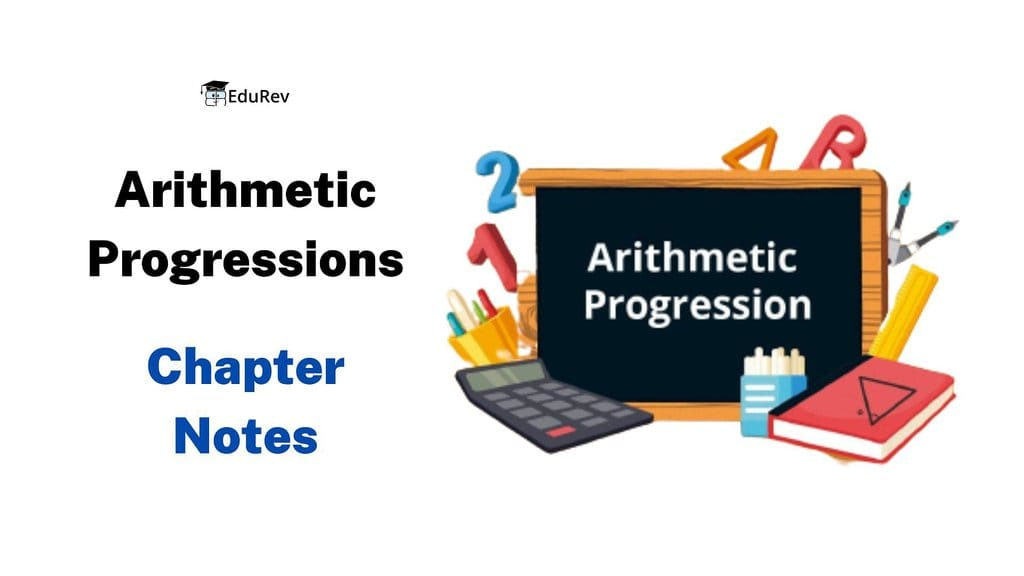In this Class 10 document, we will learn the basics of APs, their properties, practical uses, and how to solve problems related to them.

## What are Arithmetic Progressions?

Arithmetic Progressions (APs) are sequences of numbers in which the difference between consecutive terms remains constant. This constant difference is called the common difference. In an arithmetic progression, each term can be obtained by adding the common difference to the previous term.

Here is a list of numbers, observe the pattern of the numbers.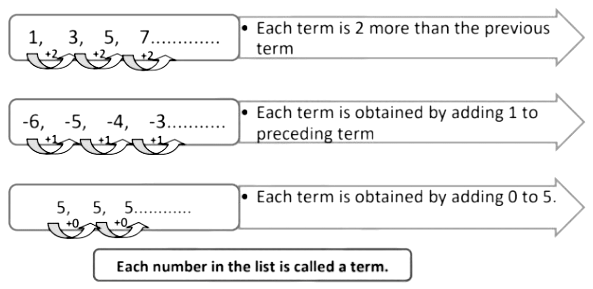• Here, we see that each successive term is obtained by adding a fixed number to the preceding term except the first term. Such a list of numbers is said to be in arithmetic progression.
• An arithmetic progression (AP) is a list of numbers in which each term is obtained by adding a fixed number to the preceding term except the first term.
This fixed number is called the common difference of the AP. This number can be positive, negative or zero.
• Let us denote the first term of an AP by a1, the second term by a2,.........nth term by an and the common difference by d. Then the AP becomes a1, a2, a3, ... ... ... ... ... an.
So, a1 − a2 = a3 − a2 =.........= an − an−1 = d

## Infinite and Finite Arithmetic Progression (AP)

Arithmetic Progressions (APs) can be categorized as either finite or infinite based on the number of terms they contain.

• Finite Arithmetic Progression: A finite Arithmetic Progression (AP) refers to a sequence of numbers that has a specific and limited number of terms. The progression stops after a certain term, and there is a finite number of terms in the sequence.
Example: 229, 329, 429, 529, 629
• Infinite Arithmetic Progression: In contrast, an infinite Arithmetic Progression (AP) is a sequence of numbers that continues indefinitely without an endpoint. It goes on infinitely, and there is no fixed limit on the number of terms. The common difference between consecutive terms remains constant throughout the progression, and the terms can be positive, negative, or zero.
Example: 2, 4, 6, 8, 10, 12, 14, 16, 18…..…

Understanding the distinction between finite and infinite APs helps us analyze different types of sequences and apply appropriate methods and formulas accordingly.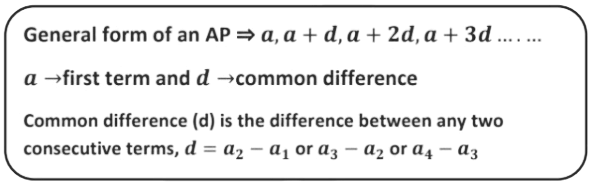### Properties of Arithmetic Progressions

• If the same number is added or subtracted from each A.P. term, the resulting terms in the sequence are also in A.P. with the same common difference.
• If each term in A.P. is divided or multiplied by the same non-zero number, the resulting series is also in A.P.
• Three numbers x, y, and z will be an A.P. if 2y = x + z.
• A series is an A.P. if the nth term is a linear expression.
• If we pick terms from the A.P. in regular intervals, these selected terms will also make an A.P.
• If the terms of an arithmetic progression are increased or decreased with the same amount, the resulting sequence will also be an arithmetic progression.

Example 1: In which of the following situations, does the list of numbers involved make an arithmetic progression, and why?

a) The cost of digging a well after every meter of digging when it costs Rs. 150 for the first meter and rises by Rs. 50 for each subsequent meter.

Sol:

Cost of digging the first meter = Rs.150
Cost of digging second metre = Rs.(150 + 50) = Rs.200
Cost of digging third metre = Rs.(150 + 2× 50) = Rs. 250
In this case, each term is obtained by adding Rs. 50 to the preceding term. Hence, they make an AP.

b) The amount of water present in a cylinder when a vacuum pump removes 1⁄4 of the air remaining in the cylinder at a time.

Sol:

Let the amount of air present in the cylinder be x units.
According to the question,
Amount of air left in the cylinder after using vacuum pump first time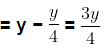Amount of air left in the cylinder after using vacuum pump the second time=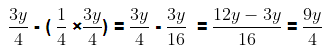List of numbers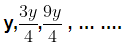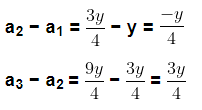As the common difference of the terms is not the same, they do not form AP.

Question for Chapter Notes: Arithmetic Progressions
Try yourself:Is the sequence {2, 5, 8, 11, 14} an arithmetic progression?

Example 2: Find the common difference of the AP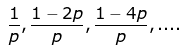Sol:
Common difference (d) = Second Term – First Term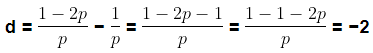Therefore, common difference = -2

Example 3: For what value of k will k + 10 , 2k, and 2k + 8 are the consecutive terms of an AP.

Sol:

If k + 10 , 2k, and 2k + 8 are in AP then,
a1 = k + 10 , a2 = 2k and a3 = 2k + 8

Common Difference (d)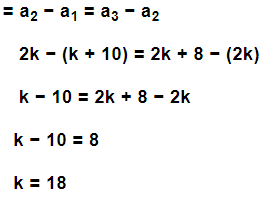Example 4: If the numbers 2n − 2, 3n + 1, and 6n − 2 are in AP,  then find n and hence find the numbers.

Sol:

The numbers 2n − 2, 3n + 1, and 6n − 2 are in AP then,
a1 = 2n − 2 , a2 = 3n + 1 and a3 = 6n − 2
Common difference (d) = a2 − a1 = a3 − a2
d = 3n + 1 − (2n − 2) = 6n − 2 − (3n + 1)
3n + 1 − 2n + 2 = 6n − 2 − 3n − 1
n + 3 = 3n − 3 ⇒ 3n − n = 3 + 3
2n = 6 ⇒ n = 3
Therefore the value of n is 3.
The numbers are,
2n − 2 = 2 × 3 − 2 = 6 − 2 = 4
3n + 1 = 3 × 3 + 1 = 9 + 1 = 10
6n − 2 = 6 × 3 − 2 = 16

Question for Chapter Notes: Arithmetic Progressions
Try yourself:What is the common difference in the arithmetic progression 5, 9, 13, 17, 21?

## nth term of an AP

Let a1 , a2, a3, ... ... ... ... ... an be an AP whose first term a1 is a and the common difference is d.

Then,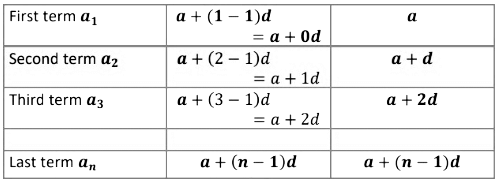The nth term an of the AP with the first term a and common difference d is given by

an = a + (n − 1)d

### Solved Examples

Example 5: Find the 25th term of the AP: -5, -5/2, 0, 5/2

Sol:

Here, a = −5
Common Difference (d) = a2 − a1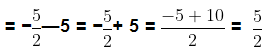We know, a= a + (n − 1)d

25th term, a25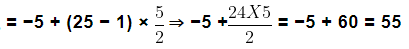25th term of the given AP is 55.

Example 6: For an AP, if a18 − a14 = 36, then find the common difference d.

Sol:

We know, an = a + (n − 1)d
a18 = a + (18 − 1)d = a + 17d
a14 = a + (14 − 1)d = a + 13d
a18 − a14 = 36
a + 17d − (a + 13d) ⇒ a + 17d − a − 13d
4d = 36
d = 9
Therefore, the common difference (d) = 9

Example 7: If an = 6 − 11n, then find the common difference.

Sol:

Given: a= 6 − 11n ............... (1)

Replacing n by n+1 in eq (1) we get

a(n+1) = 6 – 11(n+1)

a(n+1) = 6 – 11n-11

a(n+1) = - 11n – 5

Common difference, d = a(n+1) – an

d= -11n-5 – (6-11n)

d = -11

Example 8: Find the 7th term of the sequence whose nth term is given by an = (−1)n−1. n2

Sol:

Given: an = (−1)n−1. n2
The 7th term of the sequence, a7 = (−1)7−1. 72
= (−1)6. 72 = 49

Example 9: An AP consists of 50 terms of which 3rd term is 12 and the last term is 106. Find the 29th term.

Sol:

Let a be the first term and d be the common difference of the given AP.

Given: a= 12 and a50 = 106

We know, an = a + (n − 1)d
a3 = a + (3 − 1)d ⇒ a3 = a + 2d
a= a + 2d = 12→Eq 1
a50 = a + (50 − 1)d ⇒ a50 = a + 49d
a50 = a + 49d = 106→Eq 2
Subtracting Eq 1 from Eq 2

a + 49d − (a + 2d) = 106 − 12

a + 49d − a − 2d = 94

47d = 94 ⇒ d = 2

Putting the value of d in Eq 1 we get,

a + 2 × 2 = 12

a = 12 − 4 = 8

29th term, a29 = a + 28d = 8 + 28 × 2 = 8 + 56 = 64

Example 10: Find how many two-digit numbers are divisible by 7.

Sol:

Two-digit numbers are 10, 11, 12, 13, ..................97, 98, 99, 100.
Here 14, 21, 28...................... 91, 98 are divisible by 7.
This list of numbers forms an AP, where a = 14 and
d = 21 − 14 = 7
Let the number of terms be n, then an = 98

98 = 14 + (n − 1)7 ⇒ 98 = 14 + 7n − 7

7n + 7 = 98 ⇒ 7n = 98 − 7

7n = 91

n = 13
Hence, 13 two-digit numbers are divisible by 7.

Question for Chapter Notes: Arithmetic Progressions
Try yourself:In an arithmetic progression (AP), if the first term is 2 and the common difference is 4, what is the 7th term of the AP?

## Sum of first n terms of an  AP

• One of the most important aspects of AP is finding the sum of its terms. The sum of the first n terms of an AP is a commonly used formula in mathematics. It allows us to quickly find the total sum of a given number of terms in an AP without having to add each term individually.
• Sum of first n terms of an AP is given by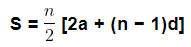• Now, this can also be written as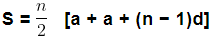• We know an = a + (n − 1)d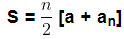• If  the number of terms in the AP is n then, an is the last term and an = I
therefore,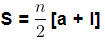• This form of the result is useful when only the first and the last term are given and the common difference is not given.

Example 11: If the nth term of an AP is (2n + 2), find the sum of first n terms of the AP.

We have,
an = 2n + 2 ⇒ a1 = 2 × 1 + 2 = 4
Therefore, a= a is the first term and an = l is the last term of the AP.
As we know the first and the last term of the AP, the sum of n terms is given by,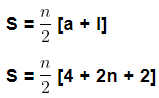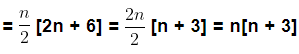Sum of first n terms of the given AP is n[n + 3]

Example 12: Find the sum of the first 25 terms of an AP, whose nth term is given by an = 6 − 3n.

Given: an = 6 − 3n
a1 = 6 − 3 × 1 = 3

a25 = 6 − 3 × 25 = −69
Therefore, a1 = a = 3 is the first term and a25 = l = −69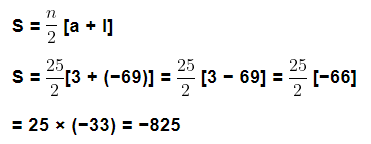Therefore, the sum of the first 25 terms of the given AP is -825.

Example 13: Find the sum of all two-digit odd positive numbers.

Two-digit odd positive numbers are 11, 13, 15..................99 which form an AP.
Here, First term a = 11, last term (l) = 99 and common difference (d) = 13 – 11 = 2
Now we have to find the number of terms.

We know, l = an = a + (n − 1)d
99 = 11 + (n − 1) × 2
99 = 11 + 2n − 2 ⇒ 99 = 9 + 2n
99 − 9 = 2n ⇒ 90 = 2n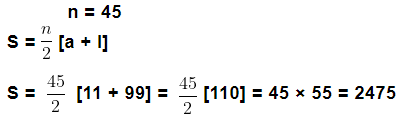Therefore, the sum of all two-digit odd positive numbers is 2475.

Question for Chapter Notes: Arithmetic Progressions
Try yourself:Find the sum of the first 10 terms of an arithmetic progression where the first term is 3 and the common difference is 5.

The document Arithmetic Progressions Chapter Notes | Mathematics (Maths) Class 10 is a part of the Class 10 Course Mathematics (Maths) Class 10.
All you need of Class 10 at this link: Class 10

## Mathematics (Maths) Class 10

115 videos|478 docs|129 tests

## FAQs on Arithmetic Progressions Chapter Notes - Mathematics (Maths) Class 10

1. What are arithmetic progressions?

Ans. Arithmetic progressions are sequences of numbers where each term is obtained by adding a fixed number to the preceding term. The fixed number is called the common difference.

2. What is the formula for finding the nth term of an arithmetic progression?

Ans. The formula for finding the nth term of an arithmetic progression is: a + (n-1)d, where a is the first term, d is the common difference, and n is the term number.

3. How do you find the sum of the first n terms of an arithmetic progression?

Ans. The formula for finding the sum of the first n terms of an arithmetic progression is: (n/2)(2a + (n-1)d), where a is the first term, d is the common difference, and n is the number of terms.

4. Can an arithmetic progression have a negative common difference?

Ans. Yes, an arithmetic progression can have a negative common difference. It means that the terms in the sequence are decreasing instead of increasing.

5. How do you find the common difference of an arithmetic progression?

Ans. To find the common difference of an arithmetic progression, subtract any term from the term that follows it.

## Mathematics (Maths) Class 10

115 videos|478 docs|129 testsExplore Courses for Class 10 examSignup to see your scores go up within 7 days! Learn & Practice with 1000+ FREE Notes, Videos & Tests.
10M+ students study on EduRev
Track your progress, build streaks, highlight & save important lessons and more!
Related Searches

,

,

,

,

,

,

,

,

,

,

,

,

,

,

,

,

,

,

,

,

,

;